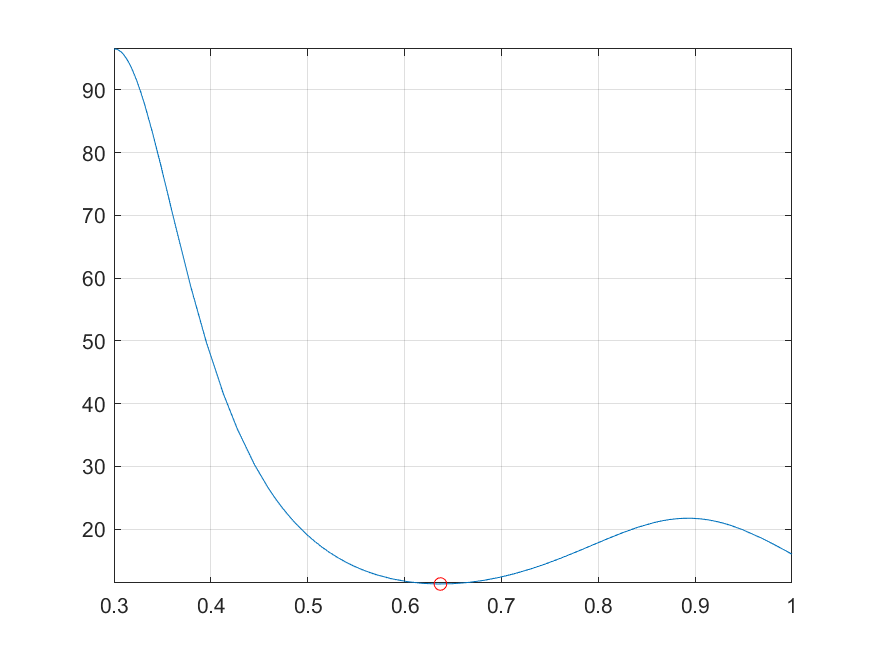## 8-4 嚙踝蕭嚙踝蕭嚙踝蕭嚙踝蕭嚙踝蕭嚙踝蕭嚙踝蕭嚙踝蕭嚙踝蕭

MATLAB 提供了數個指令來進行數學函數的最佳化，本節將介紹：

• 單變數函數的最小化
• 多變數函數的最小化
• 設定最佳化的選項

Example 1: 08-一般數學函數的處理與分析/fminbnd01.m[x, minValue] = fminbnd(@humps, 0.3, 1) % 使用 fminbnd 指令找出最小值的發生點 fplot(@humps, [0.3, 1]); grid on line(x, minValue, 'marker', 'o', 'color', 'r'); % Plot the minimum point x = 0.6370 minValue = 11.2528Example 2: 08-一般數學函數的處理與分析/fminbnd02.mopt = optimset('disp', 'iter'); % 顯示每個步驟的結果 [x, minValue] = fminbnd(@humps, 0.3, 1, opt) Func-count x f(x) Procedure 1 0.567376 12.9098 initial 2 0.732624 13.7746 golden 3 0.465248 25.1714 golden 4 0.644416 11.2693 parabolic 5 0.6413 11.2583 parabolic 6 0.637618 11.2529 parabolic 7 0.636985 11.2528 parabolic 8 0.637019 11.2528 parabolic 9 0.637052 11.2528 parabolic Optimization terminated: the current x satisfies the termination criteria using OPTIONS.TolX of 1.000000e-04 x = 0.6370 minValue = 11.2528

Example 3: 08-一般數學函數的處理與分析/fminbnd03.mopt = optimset( 'disp', 'iter', 'TolX', 0.1); % 顯示每個步驟的結果並設定誤差容忍值 [x, minValue] = fminbnd(@humps, 0.3, 1, opt) Func-count x f(x) Procedure 1 0.567376 12.9098 initial 2 0.732624 13.7746 golden 3 0.465248 25.1714 golden 4 0.644416 11.2693 parabolic 5 0.611083 11.4646 parabolic 6 0.677749 11.7353 parabolic Optimization terminated: the current x satisfies the termination criteria using OPTIONS.TolX of 1.000000e-01 x = 0.6444 minValue = 11.2693

function y = objective(x) y = (x(1)-1).^2 + 5*(x(2)-2).^2 + (x(3)-3).^2;

Example 4: 08-一般數學函數的處理與分析/fminsearch01.mx0 = [0, 0, 0]; x = fminsearch(@objective, x0) x = 1.0000 2.0000 3.0000

fminsearch 使用的方法是下坡式 Simplex 搜尋（Downhill Simplex Search），詳細參考資料可見 筆者的另一本英文著作：「Neuro – Fuzzy and Soft Computing」, Prentice Hall, 1997。

MATLAB 最佳化的方法有許多不同的選項，這些選項是經由另一個輸入引數（Input Argument）來進入 fminbnd 或 fminsearch，其使用語法為：

x = fminbnd(@function, x1, x2, options)

x = fminsearch(@function, x0, options )

options = optimset(prop1, value1, prop2, value2, …)

Example 5: 08-一般數學函數的處理與分析/optimset01.moptions = optimset('Disp', 'iter', 'TolX', 0.1); fieldNames=fieldnames(options); fprintf('No. of fields = %d\n', length(fieldNames)); options No. of fields = 55 options = <a href="matlab:helpPopup struct" style="font-weight:bold">struct</a> with fields: Display: 'iter' MaxFunEvals: [] MaxIter: [] TolFun: [] TolX: 0.1000 FunValCheck: [] OutputFcn: [] PlotFcns: [] ActiveConstrTol: [] Algorithm: [] AlwaysHonorConstraints: [] DerivativeCheck: [] Diagnostics: [] DiffMaxChange: [] DiffMinChange: [] FinDiffRelStep: [] FinDiffType: [] GoalsExactAchieve: [] GradConstr: [] GradObj: [] HessFcn: [] Hessian: [] HessMult: [] HessPattern: [] HessUpdate: [] InitBarrierParam: [] InitTrustRegionRadius: [] Jacobian: [] JacobMult: [] JacobPattern: [] LargeScale: [] MaxNodes: [] MaxPCGIter: [] MaxProjCGIter: [] MaxSQPIter: [] MaxTime: [] MeritFunction: [] MinAbsMax: [] NoStopIfFlatInfeas: [] ObjectiveLimit: [] PhaseOneTotalScaling: [] Preconditioner: [] PrecondBandWidth: [] RelLineSrchBnd: [] RelLineSrchBndDuration: [] ScaleProblem: [] SubproblemAlgorithm: [] TolCon: [] TolConSQP: [] TolGradCon: [] TolPCG: [] TolProjCG: [] TolProjCGAbs: [] TypicalX: [] UseParallel: []

Example 6: 08-一般數學函數的處理與分析/optimset02.moptions = optimset('fminbnd'); fieldNames=fieldnames(options); fprintf('No. of fields = %d\n', length(fieldNames)); for i=1:length(fieldNames) if ~isempty(options.(fieldNames{i})) fprintf('options.%s=%s\n', fieldNames{i}, num2str(options.(fieldNames{i}))); end endNo. of fields = 55 options.Display=notify options.MaxFunEvals=500 options.MaxIter=500 options.TolX=0.0001 options.FunValCheck=off

Example 7: 08-一般數學函數的處理與分析/optimset03.moptions = optimset('fminsearch'); fieldNames=fieldnames(options); fprintf('No. of fields = %d\n', length(fieldNames)); for i=1:length(fieldNames) if ~isempty(options.(fieldNames{i})) fprintf('options.%s=%s\n', fieldNames{i}, num2str(options.(fieldNames{i}))); end end No. of fields = 55 options.Display=notify options.MaxFunEvals=200*numberofvariables options.MaxIter=200*numberofvariables options.TolFun=0.0001 options.TolX=0.0001 options.FunValCheck=off

1. Display 若為 0 （預設值），則不顯示中間運算結果。反之，若不為 0，則顯示運算過程的中間結果。
2. MaxFunEvals 是函數求值運算（Function Evaluation）的最高次數，對 fminbnd 的預設值是 500，對 fminsearch 的預設值是 200 乘上 x0 的長度。
3. MaxIter 是最大疊代次數，對 fminbnd 的預設值是 500，對 fminsearch 的預設值是 200 乘上 x0 的長度。
4. TolFun 是決定終止搜尋的函數值容忍度，預設為 $10^{-4}$（此選項只被 fminsearch 用到，fminbnd 並不使用）。
5. TolX 是決定終止搜尋的自變數值容忍度，預設為 $10^{-4}$。

Hint
• 最佳化並非一蹴可及，通常其過程是一再重覆，最後才能收斂到最佳點。最佳化的結果和起始點的選定有很大的關聯；一個良好的起始點，能加快最佳化收斂的速度，並提高找到全域最佳值（Global Optimum）的機會。
• 不同版本的 MATLAB，由 optimset 指令傳回的選項可能有所不同，但是差異性並不大。

MATLAB程式設計：進階篇